Type
Quiz
Book Title
Fundamentals of Corporate Finance Standard Edition 9th Edition
ISBN 13
978-0073382395

### 978-0073382395 Chapter 3 Questions and Problems 1- 15

April 3, 2019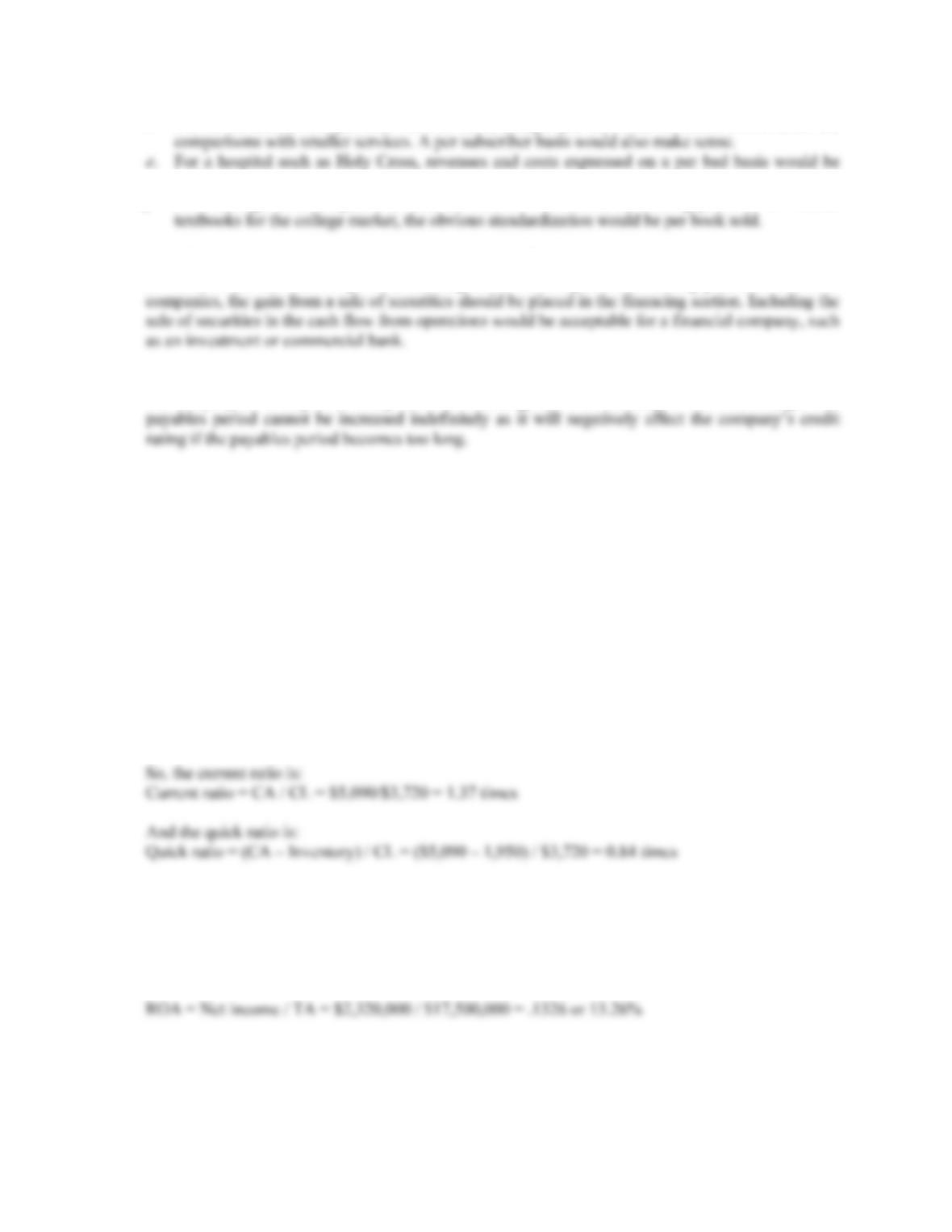CHAPTER 3 B-17
d. For an on-line service provider such as AOL, using a per call basis for costs would allow for
e. For a hospital such as Holy Cross, revenues and costs expressed on a per bed basis would be
useful.
f. For a college textbook publisher such as McGraw-Hill/Irwin, the leading publisher of finance
11. Reporting the sale of Treasury securities as cash flow from operations is an accounting “trick”, and
as such, should constitute a possible red flag about the companies accounting practices. For most
12. Increasing the payables period increases the cash flow from operations. This could be beneficial for
the company as it may be a cheap form of financing, but it is basically a one time change. The
Solutions to Questions and Problems
NOTE: All end of chapter problems were solved using a spreadsheet. Many problems require multiple
steps. Due to space and readability constraints, when these intermediate steps are included in this
solutions manual, rounding may appear to have occurred. However, the final answer for each problem is
found without rounding during any step in the problem.
Basic
1. Using the formula for NWC, we get:
NWC = CA – CL
CA = CL + NWC = \$3,720 + 1,370 = \$5,090
2. We need to find net income first. So:
Profit margin = Net income / Sales
Net income = Sales(Profit margin)
Net income = (\$29,000,000)(0.08) = \$2,320,000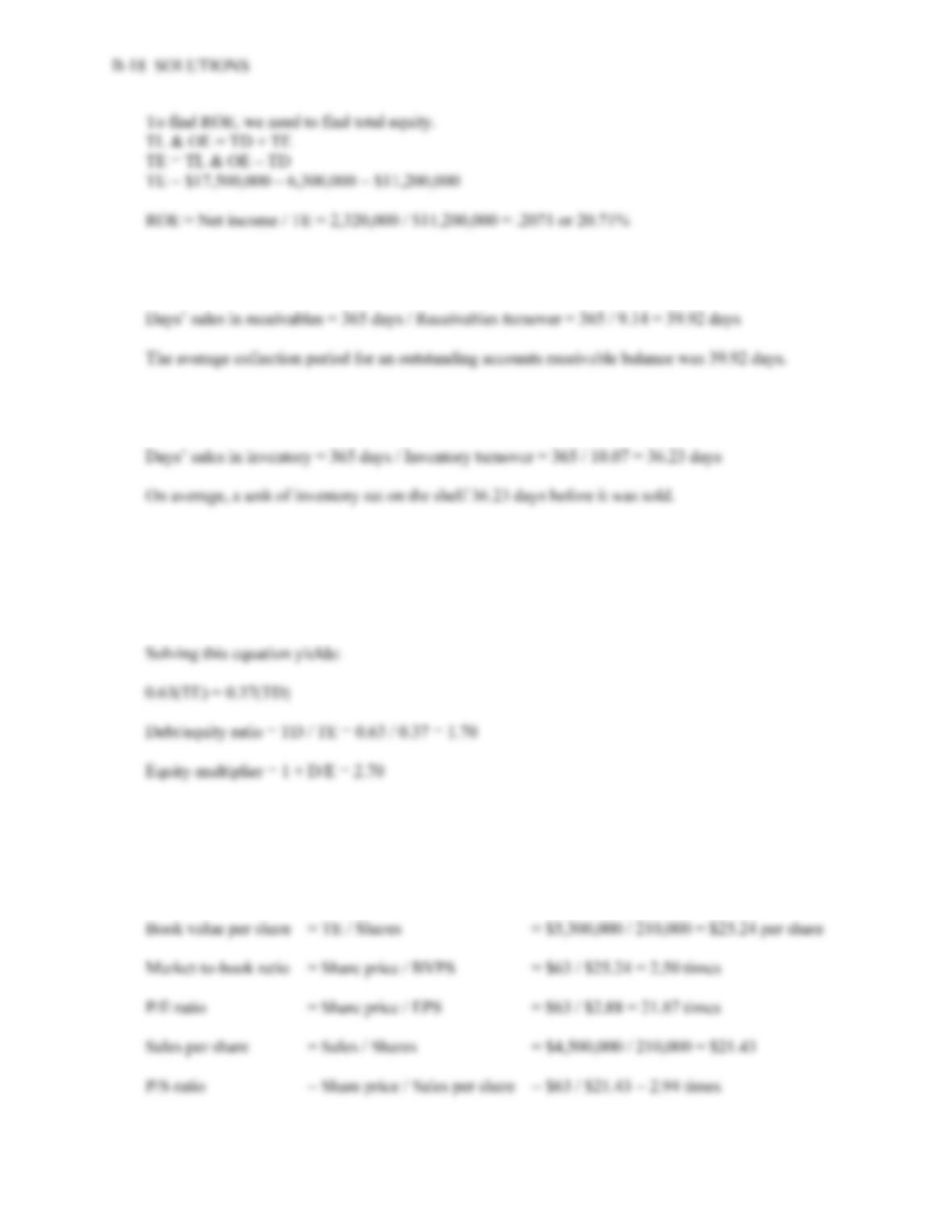3. Receivables turnover = Sales / Receivables
Receivables turnover = \$3,943,709 / \$431,287 = 9.14 times
4. Inventory turnover = COGS / Inventory
Inventory turnover = \$4,105,612 / \$407,534 = 10.07 times
5. Total debt ratio = 0.63 = TD / TA
Substituting total debt plus total equity for total assets, we get:
0.63 = TD / (TD + TE)
6. Net income = Addition to RE + Dividends = \$430,000 + 175,000 = \$605,000
Earnings per share = NI / Shares = \$605,000 / 210,000 = \$2.88 per share
Dividends per share = Dividends / Shares = \$175,000 / 210,000 = \$0.83 per share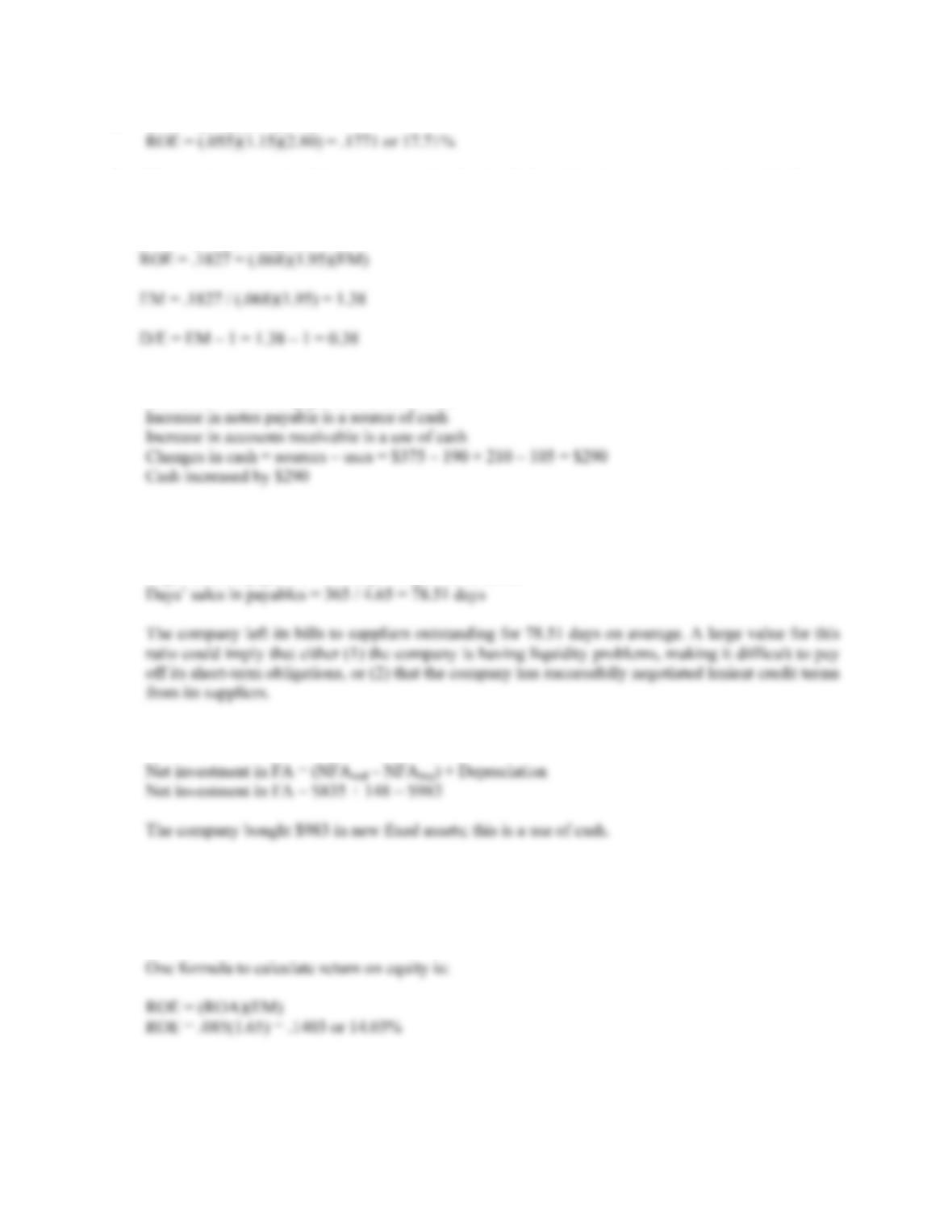CHAPTER 3 B-19
7. ROE = (PM)(TAT)(EM)
8. This question gives all of the necessary ratios for the DuPont Identity except the equity multiplier, so,
using the DuPont Identity:
ROE = (PM)(TAT)(EM)
9. Decrease in inventory is a source of cash
Decrease in accounts payable is a use of cash
10. Payables turnover = COGS / Accounts payable
Payables turnover = \$28,384 / \$6,105 = 4.65 times
Days’ sales in payables = 365 days / Payables turnover
11. New investment in fixed assets is found by:
12. The equity multiplier is:
EM = 1 + D/E
EM = 1 + 0.65 = 1.65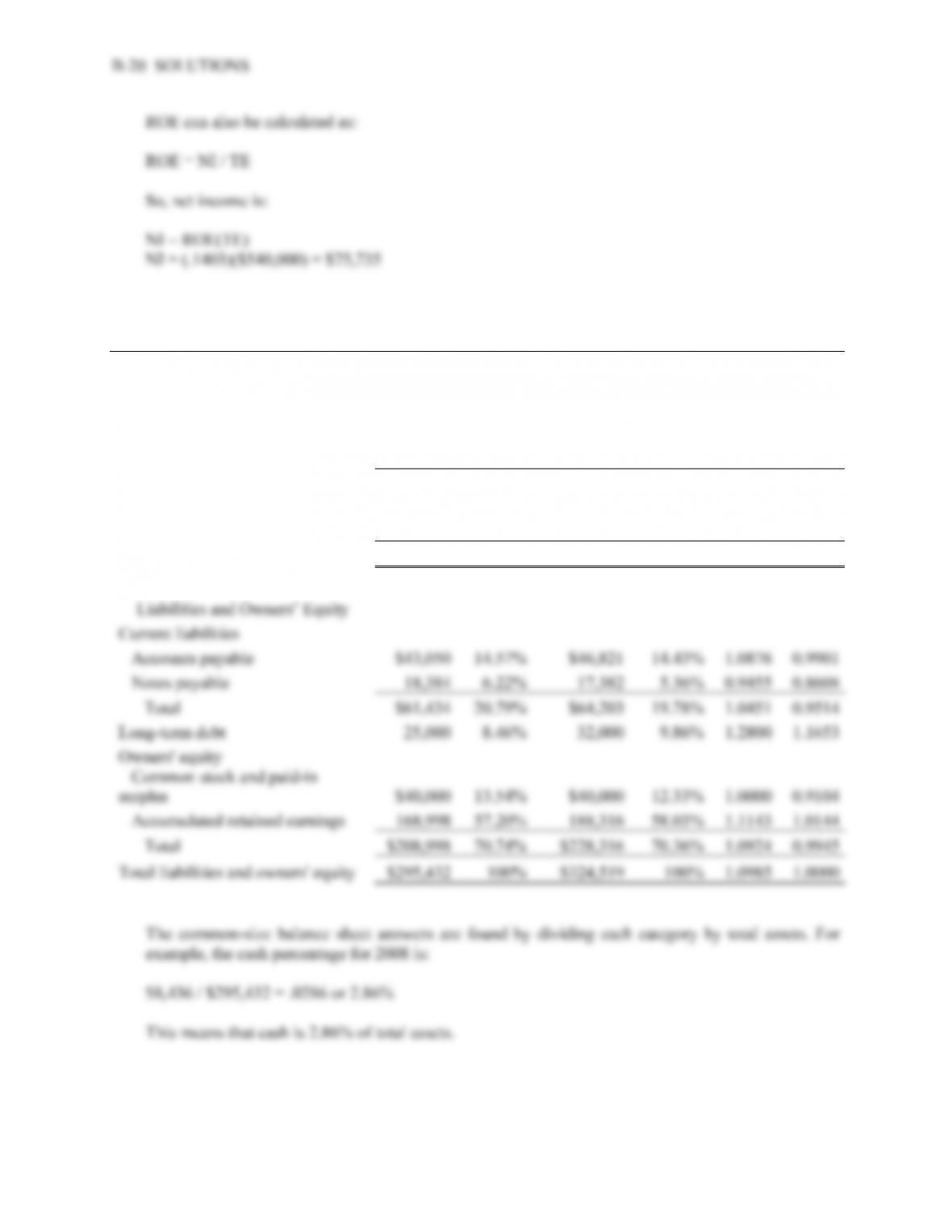13. through 15:
2008 #13 2009 #13 #14 #15
Assets
Current assets
Cash \$8,436 2.86% \$10,157 3.13% 1.2040 1.0961
Accounts receivable 21,530 7.29% 23,406 7.21% 1.0871 0.9897
Inventory 38,760 13.12% 42,650 13.14% 1.1004 1.0017
Total \$68,726 23.26% \$76,213 23.48% 1.1089 1.0095
Fixed assets
Net plant and equipment 226,706 76.74% 248,306 76.52% 1.0953 0.9971
Total assets \$295,432 100% \$324,519 100% 1.0985 1.0000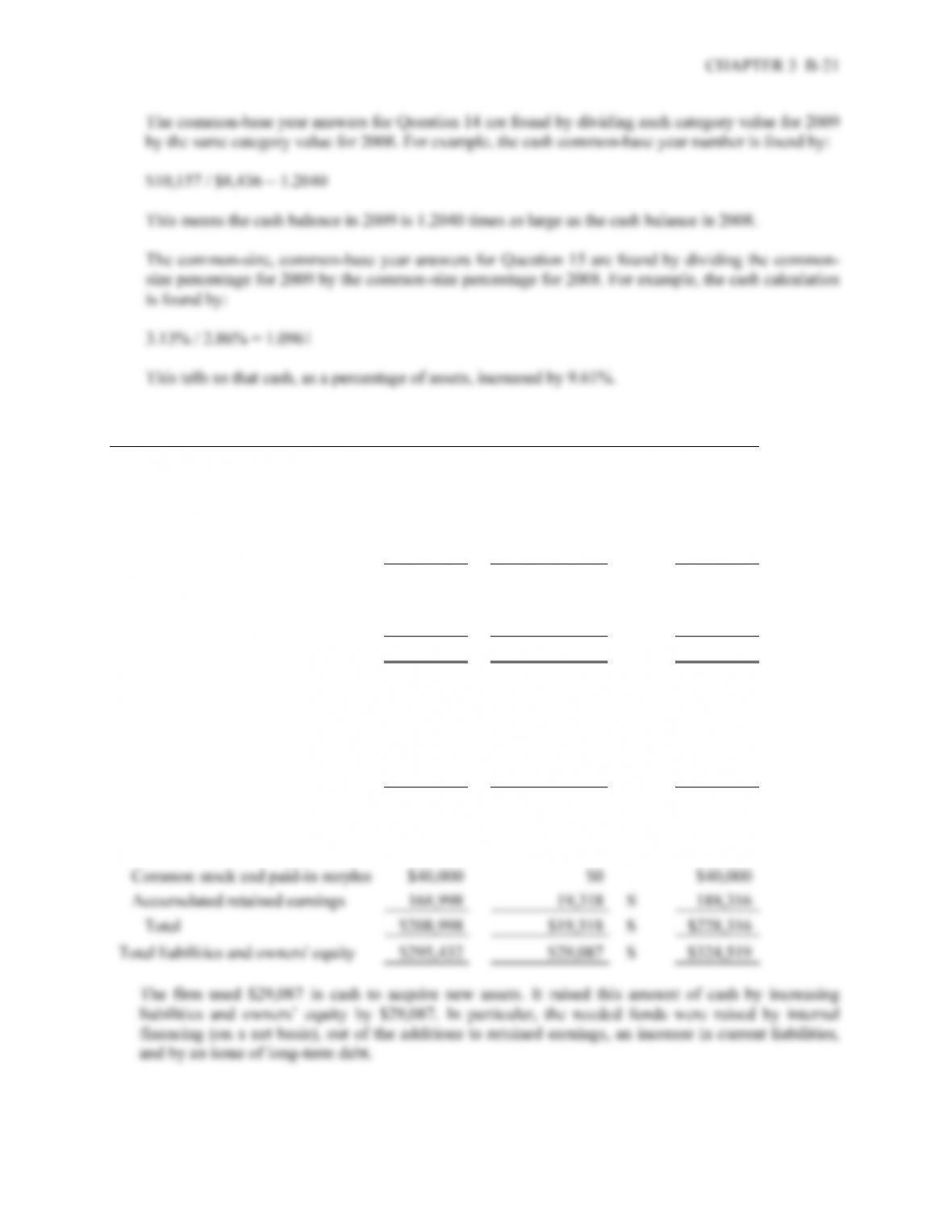16. 2008
Sources/Uses 2008
Assets
Current assets
Cash \$8,436 \$1,721 U \$10,157
Accounts receivable 21,530 1,876 U 23,406
Inventory 38,760 3,890 U 42,650
Total \$68,726 \$7,487 U \$76,213
Fixed assets
Net plant and equipment \$226,706 \$21,600 U \$248,306
Total assets \$295,432 \$29,087 U \$324,519
Liabilities and Owners’ Equity
Current liabilities
Accounts payable \$43,050 3,771 S \$46,821
Notes payable 18,384 –1,002 U 17,382
Total \$61,434 2,769 S \$64,203
Long-term debt 25,000 \$7,000 S 32,000
Owners' equity